RS Aggarwal Class 7 Solutions Chapter 5 - Exponents

RS Aggarwal Class 7 Chapter 5 - Exponents Solutions Free PDF

The amount of times a number can be used in multiplication is called as an exponent. They are also similarly referred to as Indices or Powers.The mathematical operation which involves an exponent and a base is called Exponentiation. The different types of exponents are:

1. Positive exponents
2. Negative exponents
3. Zero exponents
4. Integer exponents

A few examples of exponents are:

34 = 3 × 3 × 3 × 3 = 81

24 = 2 × 2 × 2 × 2= 16

Check out the RS Aggarwal Class 7 Solutions Chapter 5 Exponents below: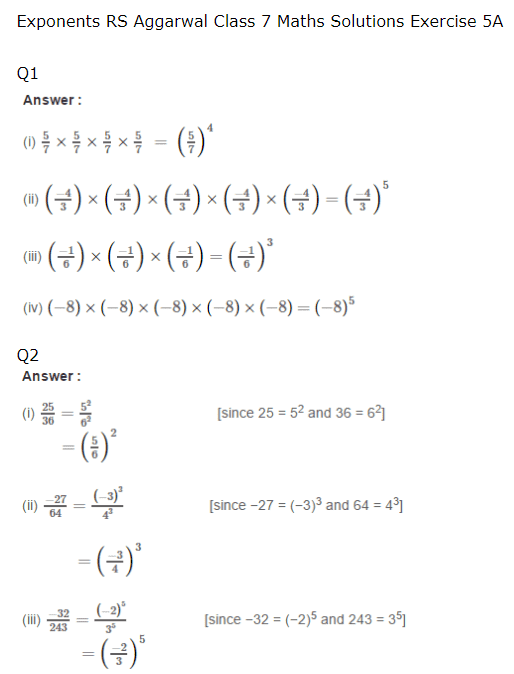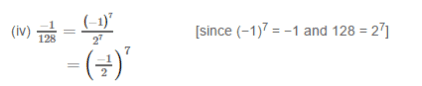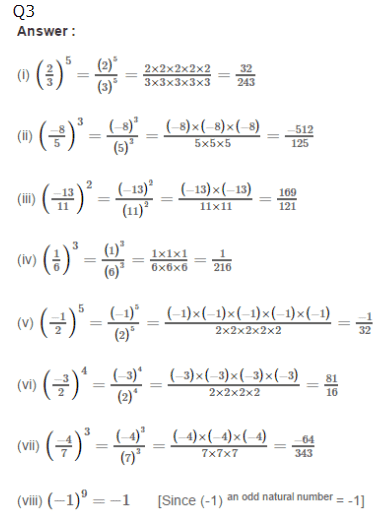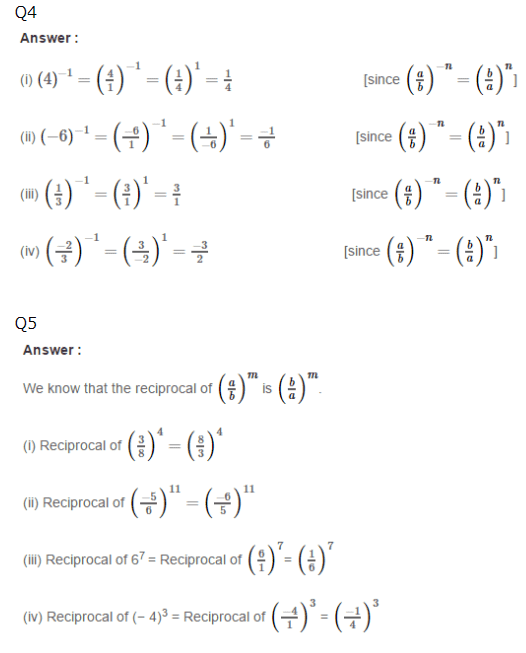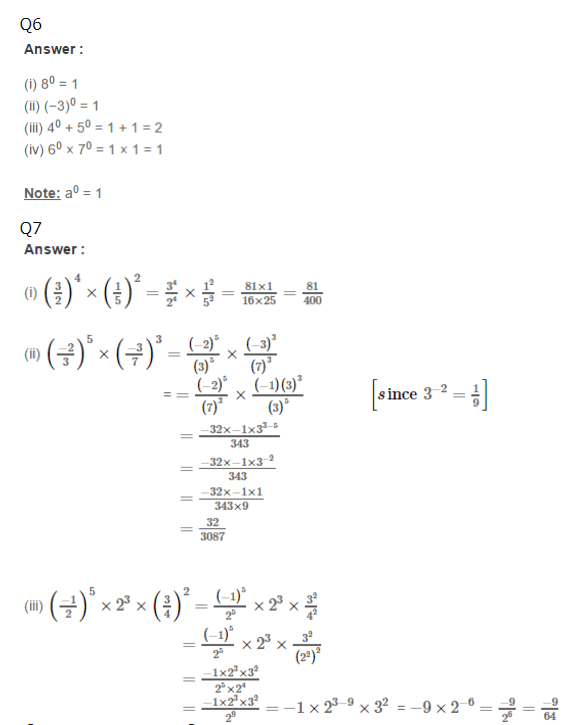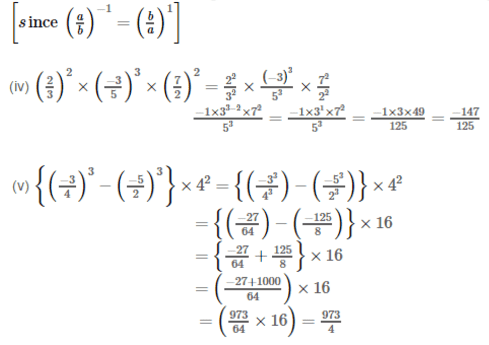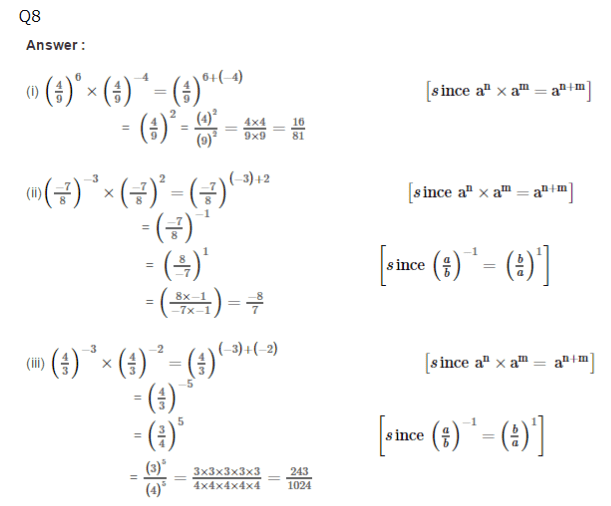Practise This Question

The endemic flora of the Pachmarhi biosphere reserve includes sal and wild mango.